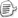VB C# Identifier(Of T) Identifier Identifier(Of T As C) Identifier where T : C

Function Add(Of T)(a As T, b As T)As T

End Function

'在另一个方法中
Dim c As Integer = Add(12)
Dim d As Double = Add(1.5888.0)

“类型字典”这个词是我根据其特性杜撰的，它其实利用到.NET泛型很重要的一个特性：泛型类的静态字段。如果有一个泛型类型A(Of T)，那么它的每一个封闭构造类型（Closed Constructed Type）独享一组静态字段的取值。也就是说，假设X是A(Of T)的静态字段（VB中的Shared或C#中的static），那么A(Of Integer).X与A(Of String).X是互相独立的两个字段，改变一个的值不会影响到另一个。这样就给我们提供了一个极为便利的条件，我们可以利用泛型类型的静态字段，为类型参数的每一组封闭取值（封闭的意思是所有取值为非泛型类型或泛型封闭构造类型）保存一个字段的值。简单地说，这就相当于以类型作为关键字，建立了一个字典，其值则是一个对象。以下为C#语法的一个小小的例子：
class TypeDict<T>
{

public static int Value;
}

//在代码中，我们可以为每个封闭类型保存一个int
//这相当于一个 类型到int 的字典
TypeDict<int>.Value = 1;
TypeDict
<string>.Value = 2;
TypeDict
<List<int>>.Value = 3;
TypeDict
<List<double>>.Value = 4;

Public Interface ICalculator(Of T)

Function Add(a As T, b As T)As TEnd Interface

Public MustInherit Class Calculator(Of T)

Implements ICalculator(Of T)

Public MustOverride Function Add(a As T, b As T) As T _

End Class

Class IntegerCalc

Inherits Calculator(Of Integer)

Public Overrides Function Add(ByVal a As IntegerByVal b As IntegerAs Integer

Return a + b

End Function
End Class

Class DoubleCalc

Inherits Calculator(Of Double)

Public Overrides Function Add(ByVal a As DoubleByVal b As DoubleAs Double

Return a + b

End Function
End Class

Class ObjectCalc(Of T)

Inherits Calculator(Of T)

Public Overrides Function Add(ByVal a As T, ByVal b As T) As T

'后期绑定的加法运算
'VB的后期绑定支持运算符重载
Return DirectCast(CObj(a) + CObj(b), T)

End Function
End Class

Public MustInherit Class Calculator(Of T)

Implements ICalculator(Of T)

Public MustOverride Function Add(ByVal a As T, ByVal b As T) As T _

'保存类型T的字典值
Private Shared defaultCalc As Calculator(Of T)

Public Shared ReadOnly Property [Default]() As Calculator(Of T)

Get

If defaultCalc Is Nothing Then CreateCalculator()

Return defaultCalc

End Get

End Property

Private Shared Sub CreateCalculator()

Dim calcObj As Object

'根据类型进行特殊子类的指派
If GetType(T).Equals(GetType(Integer)) Then
calcObj
= New IntegerCalc

ElseIf GetType(T).Equals(GetType(Double)) Then
calcObj
= New DoubleCalc

Else

'未知类型
calcObj = New ObjectCalc(Of T)

End If

'强行让编译器认为我们选择类型就是T
defaultCalc = DirectCast(calcObj, Calculator(Of T))

End Sub
End Classposted on 2006-04-14 10:12  装配脑袋  阅读(8254)  评论(7编辑  收藏  举报